# Effect of Soundproofing on Perceived Noise Levels

In this example, you measure engine noise and use psychoacoustic metrics to model its perceived loudness, sharpness, fluctuation strength, roughness, and overall annoyance level. You then simulate the addition of soundproofing material and recompute the overall annoyance level. Finally, you compare annoyance levels and show the perceptual improvements gained from applying soundproofing.

### Recording Level Calibration

Psychoacoustic measurements produce the most accurate results with a calibrated microphone input level. To use `calibrateMicrophone` to match your recording level to the reading of an SPL meter, you can use a 1 kHz tone source (such as an online tone generator or cell phone app) and a calibrated SPL meter. The SPL of the 1 kHz calibration tone should be loud enough to dominate any background noise. For a calibration example using MATLAB as the 1 kHz tone source, see `calibrateMicrophone`.

Simulate the tone recording and include some background noise. Assume an SPL meter reading of 83.1 dB (C-weighted).

```FS = 48e3; t = (1:2*FS)/FS; s = rng('default'); testTone = 0.46*sin(2*pi*t*1000).' + .1*pinknoise(2*FS); rng(s) splMeterReading = 83.1;```

To compute the calibration level of a recording chain, call `calibrateMicrophone` and specify the test tone, the sample rate, the SPL reading, and the frequency weighting of the SPL meter. To compensate for possible background noise and produce a precise calibration level, match the frequency weighting setting of the SPL meter.

`calib = calibrateMicrophone(testTone,FS,splMeterReading,"FrequencyWeighting","C-weighting");`

### Sound Pressure Levels (SPL)

Once you have a calibration factor for your recording chain, you can make acoustic measurements. When using a physical meter, you are limited to the settings selected during measurement time. With the `splMeter` object, you can change your settings after the recording has been made. This makes it easy to experiment with different time and frequency weighting options.

Load an engine recording and create the corresponding time vector.

```[x,FS] = audioread('Engine-16-44p1-stereo-20sec.wav'); x = x(1:8*FS,1); % use only channel 1 and keep only 8 seconds. t = (1:size(x,1))/FS;```

Create an `splMeter` object and select C-weighting, fast time weighting, and a 0.2 second interval for peak SPL measurement.

```spl = splMeter("CalibrationFactor",calib, ... "FrequencyWeighting","C-weighting", ... "TimeWeighting","Fast", ... "TimeInterval",0.2, ... "SampleRate",FS);```

Plot SPL and peak SPL.

```[LCF,~,LCpeak] = spl(x); plot(t,LCpeak,t,LCF) legend('LCpeak','LCF','Location',"southeast") title('SPL Measurement of Engine Noise') xlabel('Time (seconds)') ylabel('SPL (dB)') ylim([70 95]) grid on```### Psychoacoustic Metrics

#### Loudness level

Monitoring SPL is important for occupational safety compliance. However, SPL measurements do not reflect loudness as perceived by an actual listener. `acousticLoudness` measures loudness levels as perceived by a human listener with normal hearing (no hearing impairments). The `acousticLoudness` function also shows which frequency bands contribute the most to the perceptual sensation of loudness.

Using the same calibration level as before, and assuming a free-field recording (the default), plot stationary loudness.

`acousticLoudness(x,FS,calib)`The loudness is 23.8 sones, and much of the noise is below 3.3 (Bark scale). Convert 3.3 Bark to Hz using `bark2hz`

`fprintf("Loudness frequency of 3.3 Bark: %d Hz\n",round(bark2hz(3.3),-1));`
```Loudness frequency of 3.3 Bark: 330 Hz ```

The `acousticLoudness` function returns perceived loudness in sones. To understand the sone measurement, compare it to an SPL (dB) reading. A signal with a loudness of 60 phons is perceived to be as loud as a 1 kHz tone measured at 60 dB SPL. Converting 23.8 sones to phons using `sone2phon` demonstrates the loudness perception of the engine noise is as loud as a 1 kHz tone measured at 86 dB SPL.

`fprintf("Equivalent 1 kHz SPL: %d phons\n", round(sone2phon(23.8)));`
```Equivalent 1 kHz SPL: 86 phons ```

Make your own plot with units in phons and frequency in Hz on a log scale.

```[sone,spec] = acousticLoudness(x,FS,calib); barks = 0.1:0.1:24; % Bark scale for ISO 532-1 loudness hz = bark2hz(barks); specPhon = sone2phon(spec); semilogx(hz,specPhon) title('Specific Loudness') subtitle(sprintf('Loudness = %.1f phons',sone2phon(sone))) xlabel('Frequency (Hz)') ylabel('Loudness (phons/Bark)') xlim(hz([1,end])) grid on```You can also plot time-varying loudness and specific loudness to analyze the sound of the engine if it changes with time. This can be displayed with other relevant time-varying data, such as engine revolutions per minute (RPMs). In this case, the noise is stationary, but you can observe the impulsive nature of the noise.

`acousticLoudness(x,FS,calib,'TimeVarying',true,'TimeResolution','high')`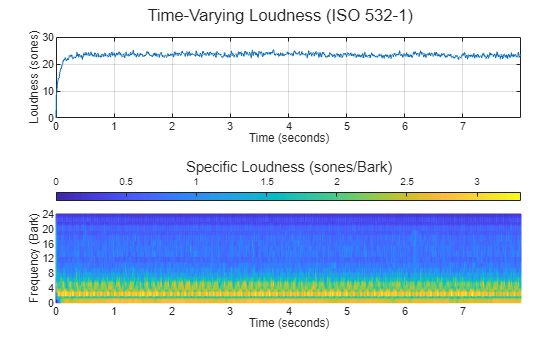Plot specific loudness with the frequency in Hz (log scale).

```[tvsoneHD,tvspecHD,perc] = acousticLoudness(x,FS,calib,'TimeVarying',true,'TimeResolution','high'); tvspec = tvspecHD(1:4:end,:,:); % for standard resolution measurements spectimeHD = 0:5e-4:5e-4*(size(tvspecHD,1)-1); % time axis for loudness output clf; % do not reuse the previous subplot surf(spectimeHD,hz,sone2phon(tvspecHD).','EdgeColor','interp'); set(gca,'View',[0 90],'YScale','log','YLim',hz([1,end])); title('Specific Loudness (HD)') zlabel('Specific Loudness (phons/Bark)') ylabel('Frequency (Hz)') xlabel('Time (seconds)') colorbar```#### Sharpness Level

The perceived sharpness of a sound can significantly contribute to its overall annoyance level. Estimate the perceived sharpness level of an acoustic signal using the `acousticSharpness` function.

`sharp = acousticSharpness(spec)`
```sharp = 1.1512 ```

Pink noise has a sharpness of 2 acums. This means the engine noise is biased towards low frequencies.

Plot time-varying sharpness.

`acousticSharpness(x,FS,calib,'TimeVarying',true);`#### Fluctuation Strength

In the case of engine noise, low-frequency modulations contribute to the perceived annoyance level.

First, look at what modulation frequencies are present in the signal.

```N = 2^nextpow2(size(x,1)); xa = abs(x); % Use the rectified signal pspectrum(xa-mean(xa),FS,'FrequencyLimits',[0 80],'FrequencyResolution',1) title('Modulation Frequencies')```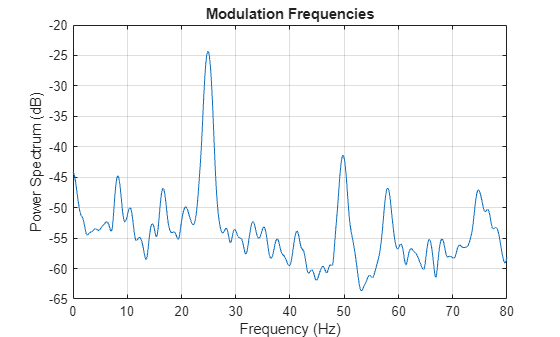The modulation frequency peaks at 24.9 Hz. Below 30 Hz, modulation is perceived dominantly as fluctuation. There is a second peak at 49.7 Hz, which is in the range of roughness.

Use `acousticFluctuation` to compute the perceived fluctuation strength. The engine noise is relatively constant in this recording, so we have the algorithm automatically detect the most audible fluctuation frequency (`fMod`).

`acousticFluctuation(x,FS,calib)`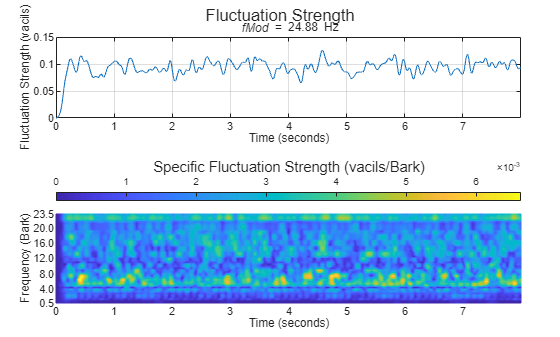Interpret the results in Hertz instead of Bark. To reduce computations, reuse the previously computed time-varying specific loudness. Alternatively, you can also specify the modulation frequency that you are interested in.

```[vacil,spec,fMod] = acousticFluctuation(tvspec,'ModulationFrequency',24.9); clf; % do not reuse previous subplot flucHz = bark2hz(0.5:0.5:23.5); spectime = 0:2e-3:2e-3*(size(spec,1)-1); surf(spectime,flucHz,spec.','EdgeColor','interp'); set(gca,'View',[0 90],'YScale','log','YLim',flucHz([1,end])); title('Specific Fluctuation Strength') zlabel('Specific Fluctuation Strength (vacils/Bark)') ylabel('Frequency (Hz)') xlabel('Time (seconds)') colorbar```#### Roughness

Use the `acousticRoughness` function to compute the perceived roughness of the signal. Let the algorithm automatically detect the most audible modulation frequency (`fMod`).

`acousticRoughness(x,FS,calib)`Interpret the results in Hertz instead of Bark. To reduce computations, reuse the previously computed time-varying specific loudness. Specify the modulation frequency.

```[asper,specR,fModR] = acousticRoughness(tvspecHD,'ModulationFrequency',49.7); clf; % do not reuse previous subplot rougHz = bark2hz(0.5:0.5:23.5); surf(spectimeHD,rougHz,specR.','EdgeColor','interp'); set(gca,'View',[0 90],'YScale','log','YLim',rougHz([1,end])); title('Specific Roughness') zlabel('Specific Roughness (aspers/Bark)') ylabel('Frequency (Hz)') xlabel('Time (seconds)') colorbar```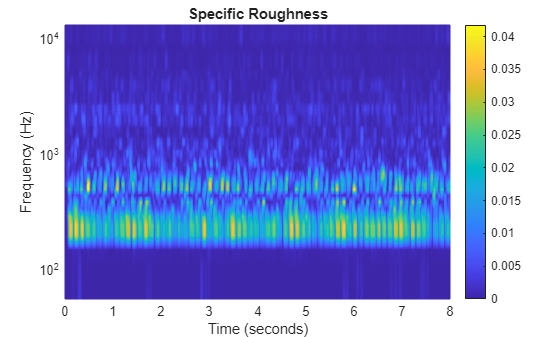### Sound Quality

For overall sound quality evaluation, combine the previous metrics to produce the psychoacoustic annoyance metric (defined by Zwicker and Fastl). The relation is as follows:

`$\mathit{PA}\sim \mathit{N}\text{\hspace{0.17em}}\left(1+\sqrt{{\left[{\mathit{g}}_{1}\left(\mathit{S}\right)\right]}^{2}+{\left[{\mathit{g}}_{2}\left(\mathit{F},\mathit{R}\right)\right]}^{2}}\right)$`

A quantitative description was developed using the results of psychoacoustic experiments:

`$\mathit{PA}={\mathit{N}}_{5}\left(1+\sqrt{{\mathit{w}}_{\mathit{S}}^{2}+{\mathit{w}}_{\mathit{FR}}^{2}}\right)$`

with:

• ${\mathit{N}}_{5}$ percentile loudness in sone (level that is exceeded only 5% of the time)

• ${\mathit{w}}_{\mathit{S}}=\left(\mathit{S}-1.75\right)\cdot \left(0.25{\cdot \mathrm{log}}_{10}\left({\mathit{N}}_{5}+10\right)\right)$ for $\mathit{S}>1.75$, where $\mathit{S}$ is the sharpness in acum

• ${\mathit{w}}_{\mathit{FR}}=\frac{2.18}{{\left({\mathit{N}}_{5}\right)}^{0.4}}\left(0.4\cdot \mathit{F}+0.6\cdot \mathit{R}\right)$, where $\mathit{F}$ is the fluctuation strength in vacil and $\mathit{R}$ is the roughness in asper

In this example, sharpness was less than 1.75, so it is not a contributing factor. Therefore, you can set ${\mathit{w}}_{\mathit{s}}$ to zero.

Percentile loudness, ${\mathit{N}}_{5}$, is the second value returned by the third output of `acousticLoudness` when `"TimeVarying"` is set to `true`.

`N5 = perc(2);`

Compute the average fluctuation strength ignoring the first second of the signal.

`f = mean(vacil(501:end,1));`

Compute the average roughness ignoring the first second of the signal.

`r = mean(asper(2001:end,1));`

Compute the psychoacoustic annoyance metric.

`pa = N5 * (1 + abs(2.18/(N5^.4)*(.4*f+.6*r)))`
```pa = 25.8848 ```

### Effect of Improved Soundproofing

Measure the impact of improved soundproofing on the measured SPL and the perceived noise.

#### Simulation Using Graphic EQ Filter Bank

Design a `graphicEQ` object to simulate the attenuation of the proposed soundproofing. Low frequencies are harder to attenuate, so we create a model that is best above 200 Hz.

```geq = graphicEQ("Bandwidth","1 octave","SampleRate",FS,"Gains",[-0.5 -1.25 -3.4 -7 -8.25 -8.4 -8 -7 -6.4 -5.6]); cf = getCenterFrequencies(splMeter("Bandwidth","1 octave"));```

Display the frequency response of the `graphicEQ` object.

```[B,A] = coeffs(geq); sos = [B;A].'; [H,w] = freqz(sos,2^16,FS); semilogx(w,db(abs(H))) title('Frequency Response of Soundproofing Simulation Filter') ylabel('Relative SPL (dB)') xlabel('Frequency (Hz)') xlim(cf([1,end])) grid on```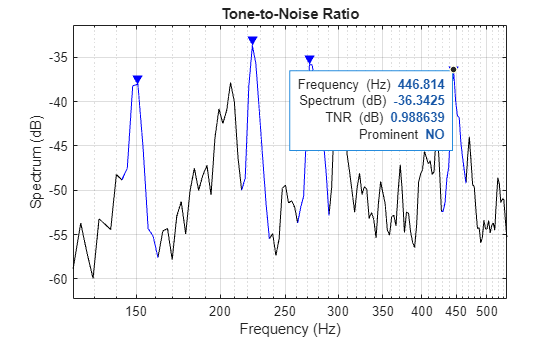Filter the engine recording using the graphic EQ to simulate the soundproofing.

`x2 = geq(x);`

Compare the SPL with and without soundproofing. Reuse the same SPL meter settings, but use the filtered recording.

```reset(spl) [LCFnew,~,LCpeaknew] = spl(x2); plot(t,LCpeak,t,LCF,t,LCpeaknew,t,LCFnew) legend('LCpeak (original)', 'LCF (original)', ... 'LCpeak (with soundproofing)', ... 'LCF (with soundproofing)', ... 'Location','southeast') title('SPL Measurement of Engine Noise') xlabel('Time (seconds)') ylabel('SPL (dB)') ylim([70 95]) grid on```Compare the perceived loudness measurements before and after soundproofing.

`acousticLoudness(x2,FS,calib)`Loudness decreased from 23.8 to 16.3 sones. However, it might be easier to interpret loudness in phons. Convert the sone units to phons using `sone2phon`.

`fprintf("Loudness without soundproofing: \t%.1f phons\n",sone2phon(23.8));`
```Loudness without soundproofing: 85.7 phons ```
`fprintf("Loudness with added soundproofing:\t%.1f phons\n",sone2phon(16.3));`
```Loudness with added soundproofing: 80.3 phons ```
`fprintf("Perceived noise reduction:\t\t%.1f phons (dB SPL at 1 kHz)\n",sone2phon(23.8)-sone2phon(16.3));`
```Perceived noise reduction: 5.5 phons (dB SPL at 1 kHz) ```

After soundproofing, `acousticLoudness` shows the perception of the engine noise is approximately 5.5 dB quieter. Human perception of sound is limited at very low frequencies, where most of the engine noise is. The soundproofing is more effective at higher frequencies.

Calculate the reduction in the psychoacoustic annoyance factor. Start by computing the mean of the acoustic sharpness.

```[~,spec2hd,perc2] = acousticLoudness(x2,FS,calib,"TimeVarying",true,"TimeResolution","high"); spec2 = spec2hd(1:4:end,:,:); shp = acousticSharpness(spec2,'TimeVarying',true); new_sharp = mean(shp(501:end))```
```new_sharp = 1.0796 ```

Sharpness has decreased because the soundproofing is more effective at high frequency attenuation. It is below the threshold of 1.75, so it is ignored for the annoyance factor.

Now, compute the mean of fluctuation strength and roughness.

```vacil2 = acousticFluctuation(spec2); f2 = mean(vacil2(501:end,1)); asper2 = acousticRoughness(spec2hd); r2 = mean(asper2(2001:end,1));```

Compute the new psychoacoustic annoyance factor. It has decreased, from 25.9 to 17.7.

```N5hp = perc2(2); % N5 with soundproofing pahp = N5hp * (1 + abs(2.18/(N5hp^.4)*(.4*f2+.6*r2)))```
```pahp = 17.7364 ```

### References

 Zwicker, Eberhard, and Hugo Fastl. Psychoacoustics: Facts and Models. Vol. 22. Springer Science & Business Media, 2013.